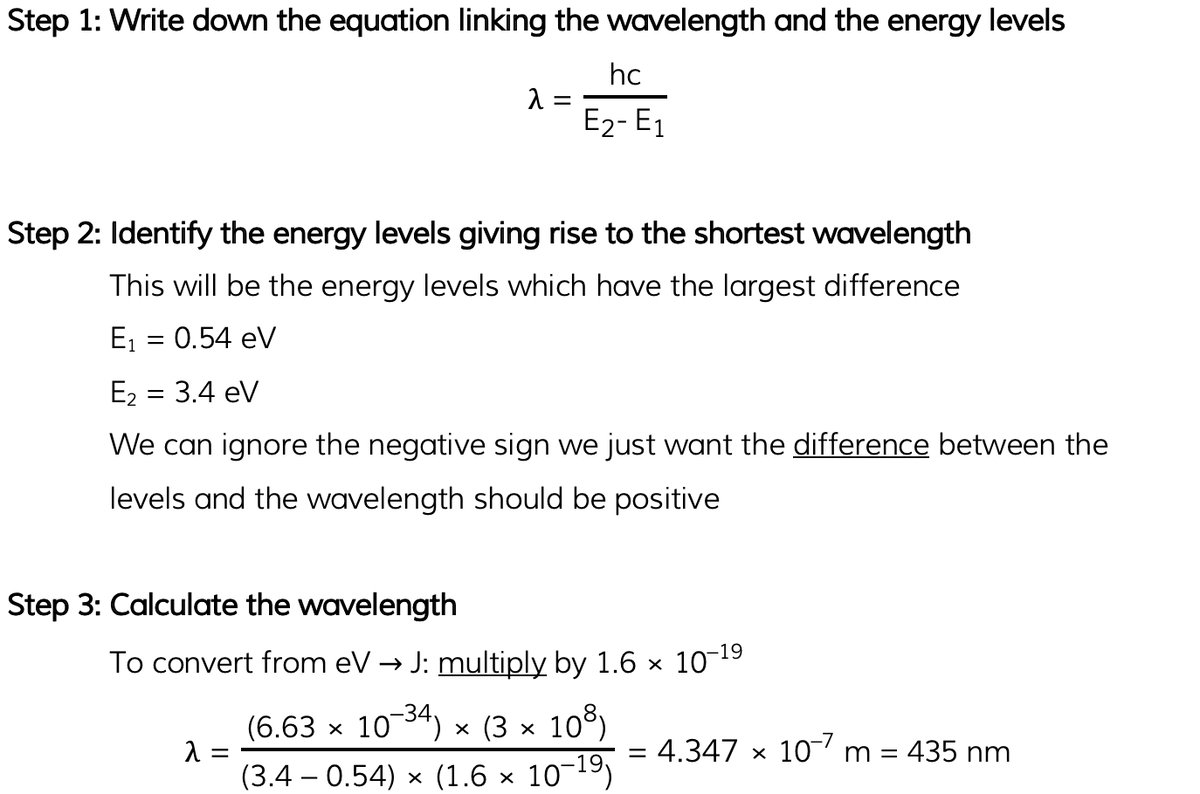# AQA A Level Physics复习笔记2.5.2 Energy Levels & Photon Emission

### Line Spectra & Energy Levels

#### Atomic Energy Levels

• Electrons in an atom can have only certain specific energies
• These energies are called electron energy levels

• They can be represented as a series of stacked horizontal lines increasing in energy
• Normally, electrons occupy the lowest energy level available, this is known as the ground state
• Electrons can gain energy and move up the energy levels if it absorbs energy either by:
• Collisions with other atoms or electrons
• Absorbing a photon
• A physical source, such as heat

• This is known as excitation, and when electrons move up an energy level, they are said to be in an excited state
• If the electron gains enough energy to be removed from the atom entirely, this is known as ionisation
• When an electron returns to a lower energy state from a higher excited state, it releases energy in the form of a photon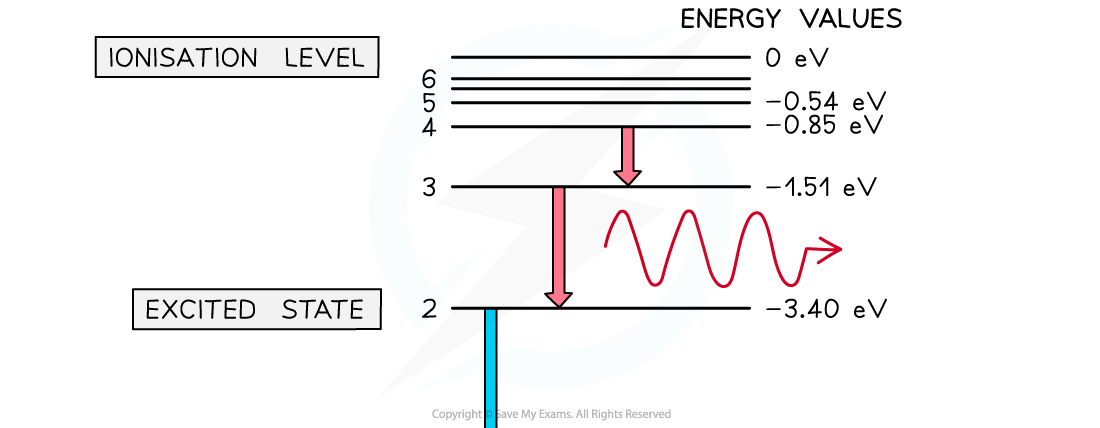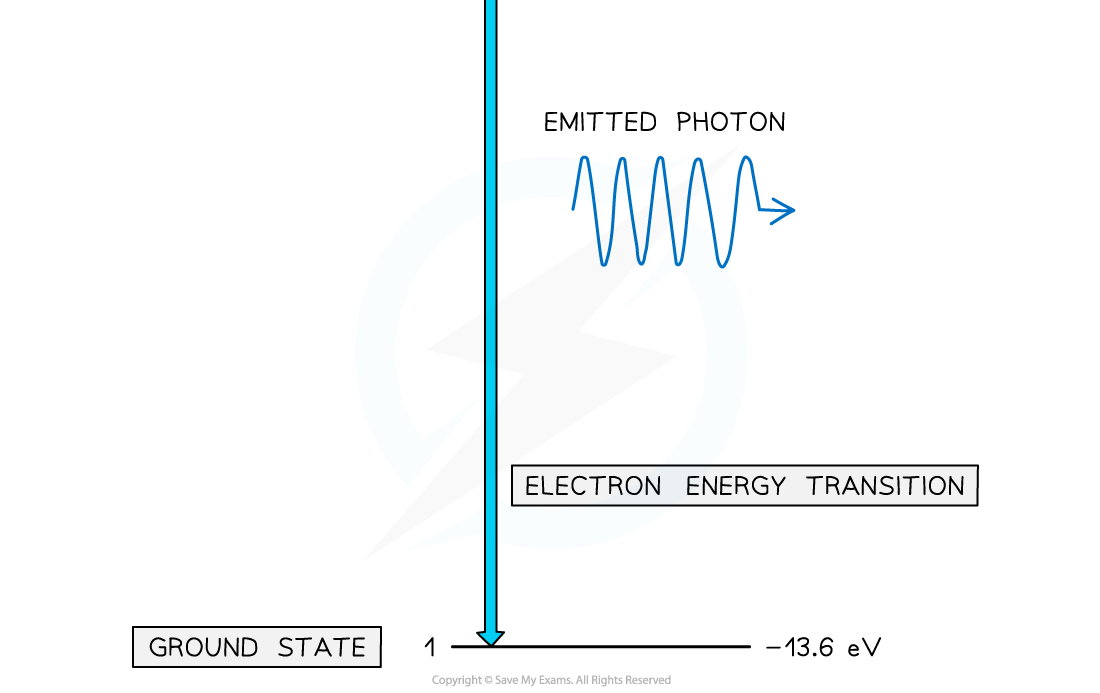Electron energy levels in atomic hydrogen. Photons are emitted when an electron moves from a higher energy state to a lower energy state

#### Line Spectra

• Line spectra is a phenomenon which occurs when excited atoms emit light of certain wavelengths which correspond to different colours
• The emitted light can be observed as a series of coloured lines with dark spaces in between
• These series of coloured lines are called line or atomic spectra

• Each element produces a unique set of spectral lines
• No two elements emit the same set of spectral lines, therefore, elements can be identified by their line spectrum
• There are two types of line spectra: emission spectra and absorption spectra

#### Emission Spectra

• When an electron transitions from a higher energy level to a lower energy level, this results in the emission of a photon
• Each transition corresponds to a different wavelength of light and this corresponds to a line in the spectrum
• The resulting emission spectrum contains a set of discrete wavelengths, represented by coloured lines on a black background
• Each emitted photon has a wavelength which is associated with a discrete change in energy, according to the equation:• Where:
• ΔE = change in energy level (J)
• h = Planck’s constant (J s)
• f = frequency of photon (Hz)
• c = the speed of light (m s-1)
• λ = wavelength of the photon (m)

• Therefore, this is evidence to show that electrons in atoms can only transition between discrete energy levels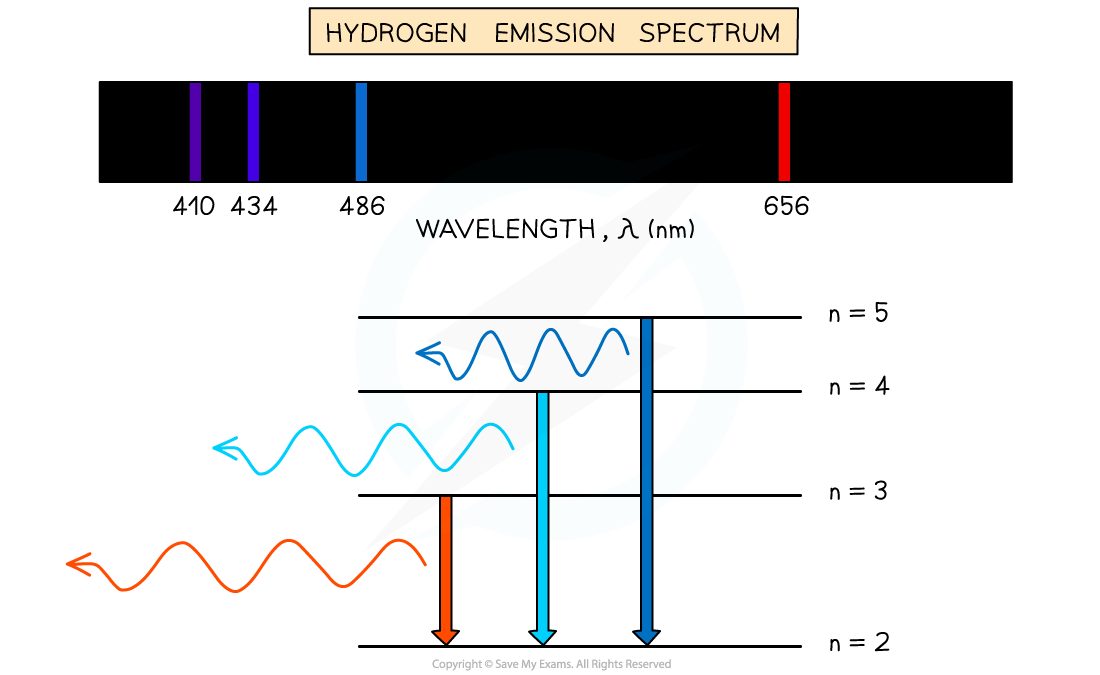Emission spectrum of Hydrogen gas

#### Absorption Spectra

• An atom can be raised to an excited state by the absorption of a photon
• When white light passes through a cool, low pressure gas it is found that light of certain wavelengths are missing
• This type of spectrum is called an absorption spectrum

• An absorption spectrum consists of a continuous spectrum containing all the colours with dark lines at certain wavelengths
• These dark lines correspond exactly to the differences in energy levels in an atom
• When these electrons return to lower levels, the photons are emitted in all directions, rather than in the original direction of the white light
• Therefore, some wavelengths appear to be missing

• The wavelengths missing from an absorption spectrum are the same as their corresponding emission spectra of the same element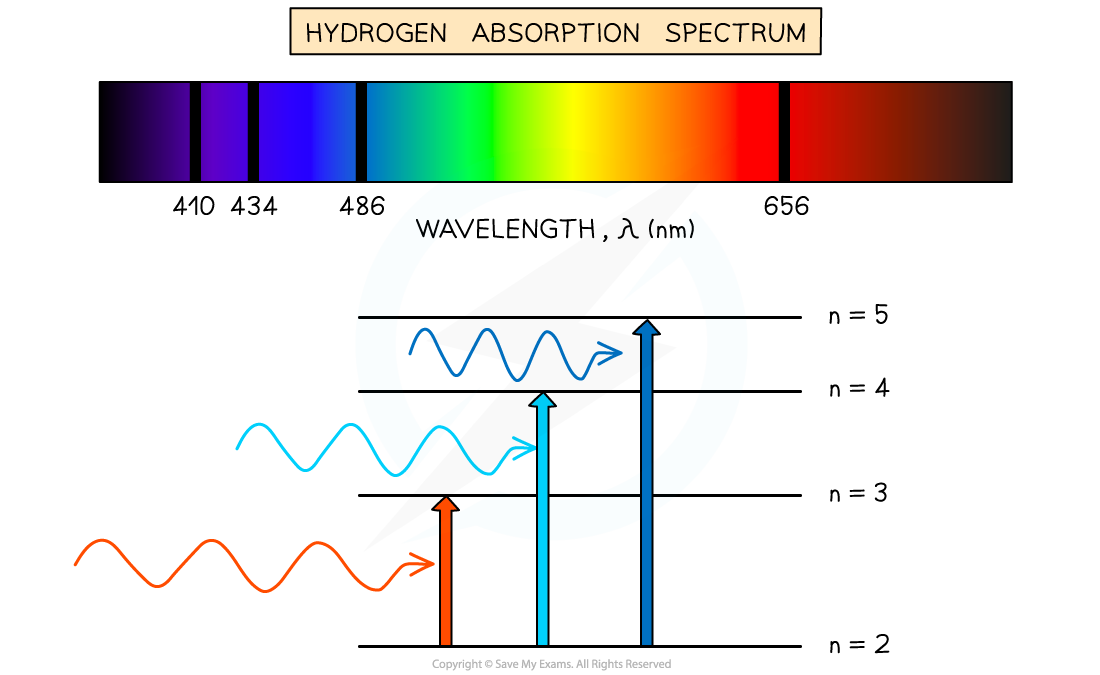Absorption spectrum of Hydrogen gas

### Difference in Discrete Energy Levels

• The difference between two energy levels is equal to a specific photon energy
• The energy (hf) of the photon is given by:

ΔE = hf = E2 - E1

• Where:
• E1 = Energy of the higher level (J)
• E2 = Energy of the lower level (J)
• h = Planck’s constant (J s)
• f = Frequency of photon (Hz)

• Using the wave equation, the wavelength of the emitted, or absorbed, radiation can be related to the energy difference by the equation:• This equation shows that the larger the difference in energy of two levels ΔE (E2 - E1) the shorter the wavelength λ and vice versa

#### Worked Example

Some electron energy levels in atomic hydrogen are shown below.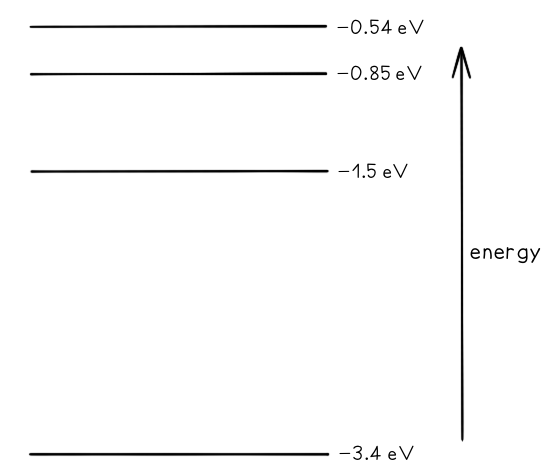The longest wavelength produced as a result of electron transitions between two of the energy levels is 4.0 × 10–6 m.a) Draw and mark:

• The transition giving rise to the wavelength of 4.0 × 10–6 m with letter L.
• The transition giving rise to the shortest wavelength with letter S.

b) Calculate the wavelength for the transition giving rise to the shortest wavelength.

Part (a)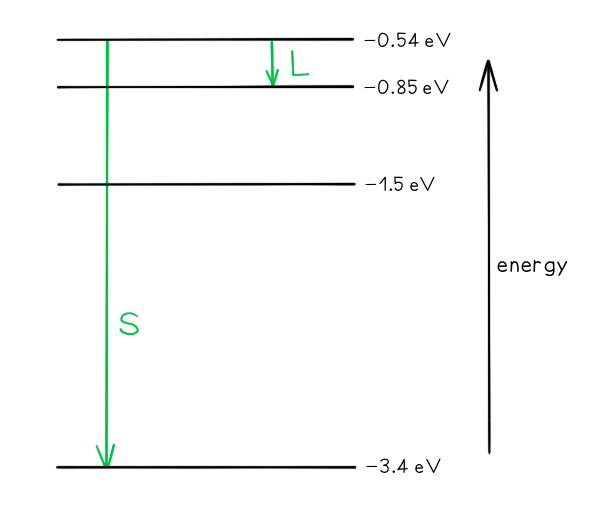• Photon energy and wavelength are inversely proportional
• Therefore, the largest energy change corresponds to the shortest wavelength (line S)
• The smallest energy change corresponds to the longest wavelength (line L)

Part (b)